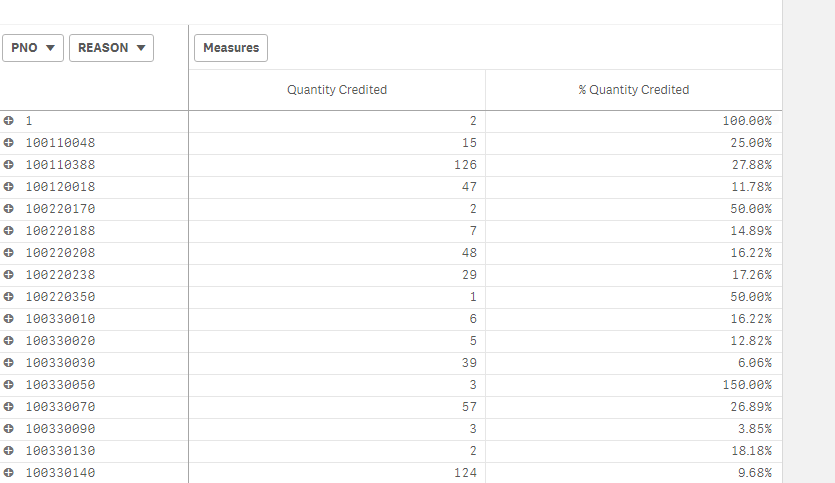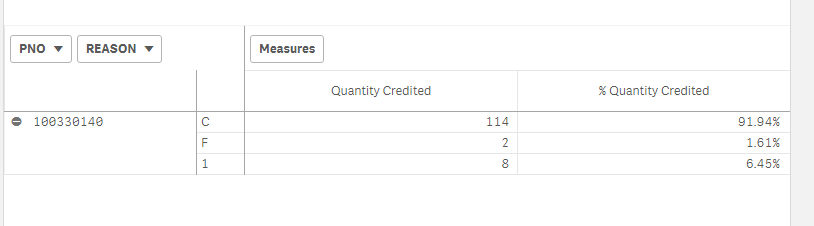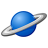# QlikView App Dev

Discussion Board for collaboration related to QlikView App Development.

Announcements
Skip the ticket, Chat with Qlik Support instead for instant assistance.
cancel
Showing results for
Did you mean:Contributor

## How to show percentage of subtotal in pivot table?

I am trying to show percentage in pivot table in Qlik sense.Quantity credit is how many numbers of a particular PNO are returned. For example, out of total sales for PNO 100330140, the last one, 124 are returned for various reasons.

the percentage returned is 9.68%, that means 1281 parts were sold before taking credits into account.

The next level is reason which tells us out of 9.68% how many were returned for different reasons.This is what i wanted without selecting a part number, but on the pivot table itself. But when I expand pivot table for rows, I don't get desired results. I am getting percentage for whole data. Here I need 91.94%, 1.61%, and 6.45% for reasons C,F, and I respectively.

What I am getting is below :Formulae I am using for second measure (%Quantity Credited) is :

if(Dimensionality() <> 2,

fabs(Sum({<ORDTYPE={"CR"}>}TotalOrderQuantity))/sum({<ORDTYPE={"SL"}>}TotalOrderQuantity),

fabs(Sum({<ORDTYPE={"CR"}>}TotalOrderQuantity))/fabs(Sum(total{<ORDTYPE={"CR"}>}TotalOrderQuantity)))

Please let me know how to get desired result.

Thanks,

Vivek

1 Solution

Accepted SolutionsSpecialist

It should be

if(Dimensionality() <> 2,

fabs(Sum({<ORDTYPE={"CR"}>}TotalOrderQuantity))/sum({<ORDTYPE={"SL"}>}TotalOrderQuantity),

fabs(Sum({<ORDTYPE={"CR"}>}TotalOrderQuantity))/fabs(Sum(total <PNO> {<ORDTYPE={"CR"}}TotalOrderQuantity)))

4 RepliesSpecialist

You are using TOTAL which will ignore all dimensions, but you should ignore the first dimension:

if(Dimensionality() <> 2,

fabs(Sum({<ORDTYPE={"CR"}>}TotalOrderQuantity))/sum({<ORDTYPE={"SL"}>}TotalOrderQuantity),

fabs(Sum({<ORDTYPE={"CR"}>}TotalOrderQuantity))/fabs(Sum(total <first_Dim> {<ORDTYPE={"CR"}>}TotalOrderQuantity)))Contributor
Author

I used the following expression as well :

if(Dimensionality() <> 2,

fabs(Sum({<ORDTYPE={"CR"}>}TotalOrderQuantity))/sum({<ORDTYPE={"SL"}>}TotalOrderQuantity),

fabs(Sum({<ORDTYPE={"CR"}>}TotalOrderQuantity))/fabs(Sum(total PNO{<ORDTYPE={"CR"}}TotalOrderQuantity)))

It doesn't work.The thing is this work when I am selecting a part number.  the percentages are correct.  I believe there is an issue in aggregate because after selecting one part number, aggregate would be same.

Thanks,

VivekSpecialist

It should be

if(Dimensionality() <> 2,

fabs(Sum({<ORDTYPE={"CR"}>}TotalOrderQuantity))/sum({<ORDTYPE={"SL"}>}TotalOrderQuantity),

fabs(Sum({<ORDTYPE={"CR"}>}TotalOrderQuantity))/fabs(Sum(total <PNO> {<ORDTYPE={"CR"}}TotalOrderQuantity)))Contributor
Author

Thanks RakeshCommunity Browser